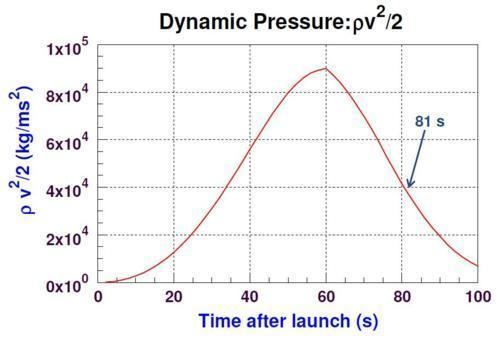# Max Q

Updated on
Covid-19## Kerbal physics 101 max q dynamic pressure

In aerospace engineering, the maximum dynamic pressure, often referred to as maximum Q or max Q, is the point at which aerodynamic stress on a vehicle in atmospheric flight is maximized. It is an important factor in the structural and mission design of rockets, missiles, and other aerospace vehicles which travel through an atmosphere; the flight envelope may be limited to reduce the total structural load on a vehicle near max Q.

## Contents

Dynamic pressure, q, is defined mathematically as

q = 1 2 ρ v 2 ,

where ρ is the local air density, and v is the vehicle's velocity; the dynamic pressure can be thought of as the kinetic energy density of the air with respect to the vehicle. For a launch of a rocket from the ground into space, dynamic pressure is

• zero at lift-off, when the air density ρ is high but the vehicle's speed v = 0
• zero outside the atmosphere, where the speed v is high, but the air density ρ = 0
• always non-negative, given the quantities involved
• Therefore (by Rolle's theorem) there will always be a point where the dynamic pressure is maximum. The point where that occurs is max Q.

In other words, before reaching max Q, the dynamic pressure change due to increasing velocity is greater than that due to decreasing air density so that the dynamic pressure (opposing kinetic energy) acting on the craft continues to increase. After passing max Q, the opposite is true. The dynamic pressure acting against the craft decreases as the air density decreases, ultimately reaching 0 when the air density becomes zero.

During a normal Space Shuttle launch, for example, max Q occurred at an altitude of approximately 11 km (35,000 ft). The three Space Shuttle Main Engines were throttled back to about 60-70% of their rated thrust (depending on payload) as the dynamic pressure approached max Q; combined with the propellant perforation design of the solid rocket boosters, which reduced the thrust at max Q by one third after 50 seconds of burn, the total stresses on the vehicle were kept to a safe level.

During a typical Apollo mission, max Q occurred between 13 and 14 km of altitude (43,000–46,000 ft).

The point of max Q is a key milestone during a rocket launch, as it is the point at which the airframe undergoes maximum mechanical stress.

## Rocket sciencee 101 max q and how to get to orbit

Max Q Wikipedia

Topics